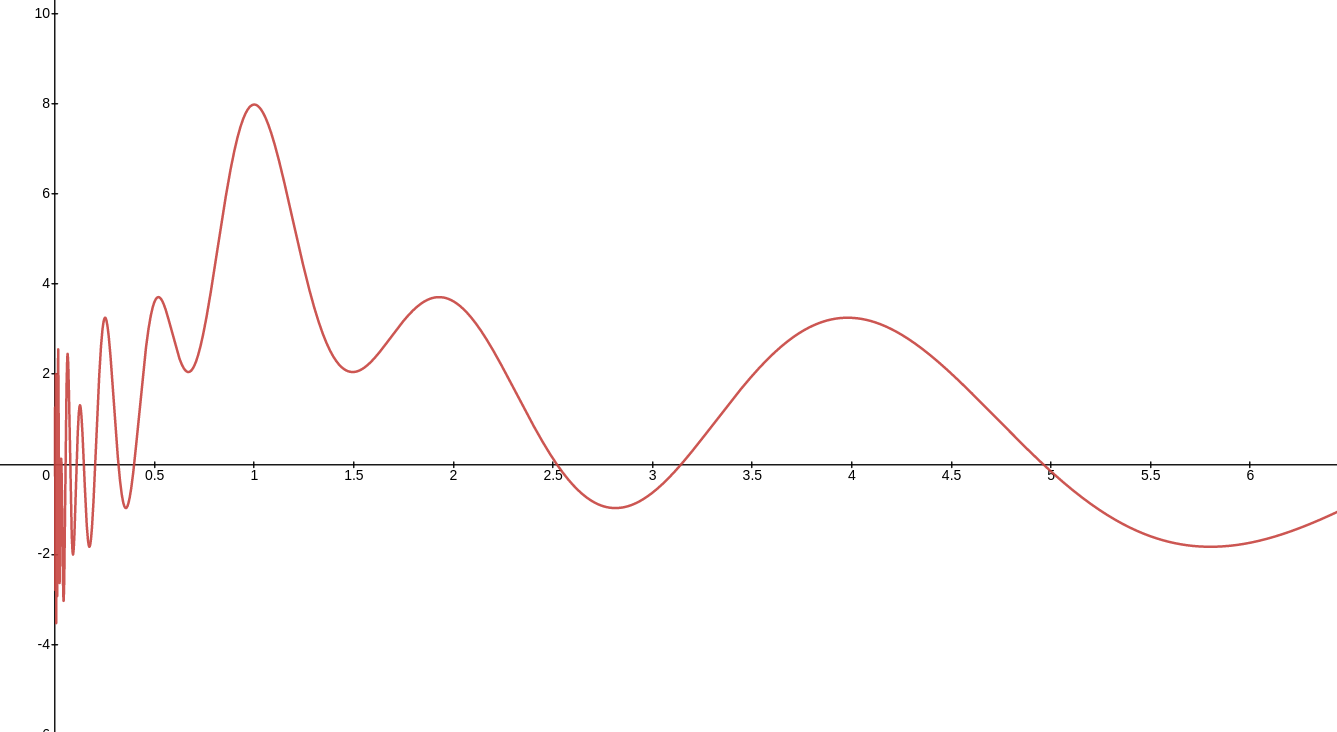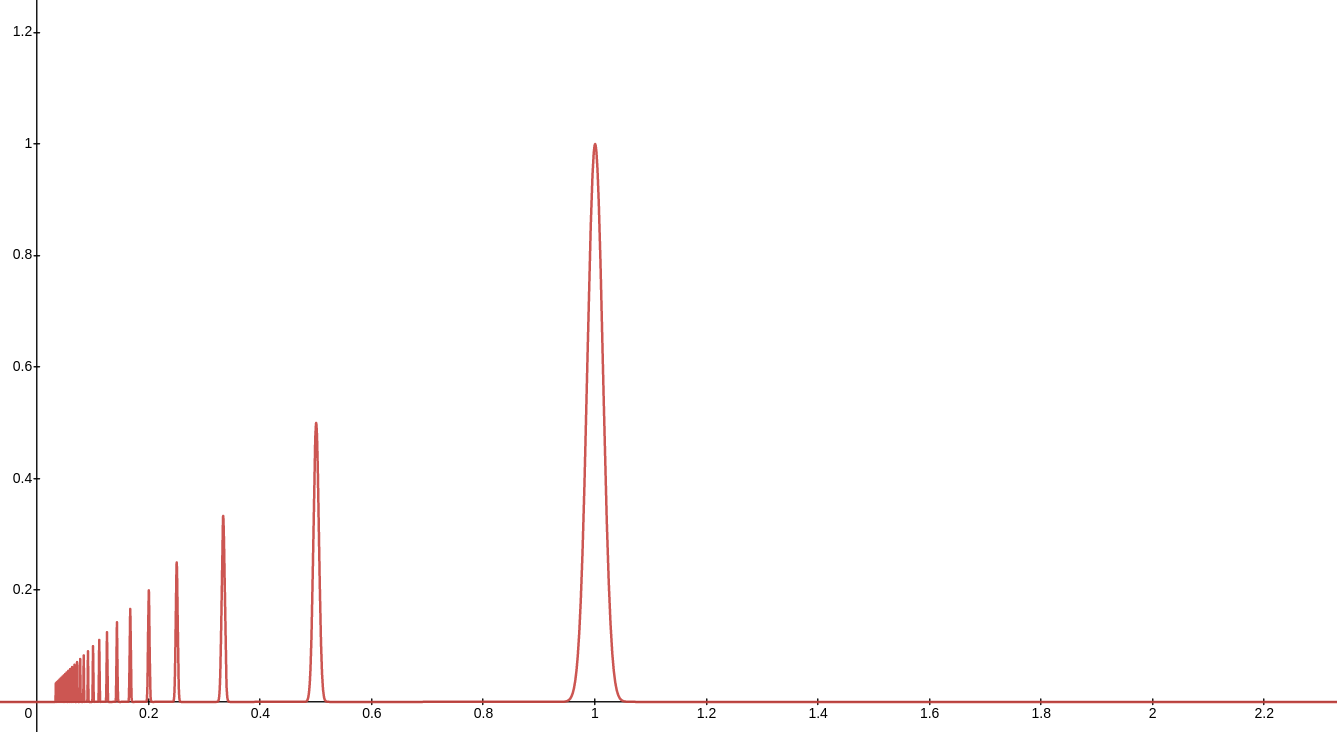# Harmonicity function

I've been struggling to create a function that returns how harmonic a frequency is in relation to some other frequency; or in other words, a function of harmonicity of a frequency.

Many sources mention the harmonic series. According to this model, we can find the most harmonic frequencies in sequency by dividing the frequency over incrementing integer numbers (i.e. 440 * 1/2, 440 * 1/3, 440 * 1/4, 440 * 1/5 and so on). The greater the denomitator is, the less harmonic the frequency is found to be in relation to the initial frequency.

There is one problem with the harmonic series, though: it doesn't take into account that frequencies that are close enough numerically, also tend to cause a perception of harmony. This is the underlying principle under equal temperament: although the frequencies in this tuning system are not harmonic, they are close enough to fool most listeners into perceiving the intended frequencies as the same. Each listener might have a different level of tonal discrimination capacity. The same goes for physical systems.

I decided to use the sine function, because thanks to it's periodicity, it is easy to create a function that already represents the harmonicity of a tone and it's octave: y = cos(2 pi x).

The logic second step is integrating a function to represent the second and third harmonics. Each harmonic should be multiplied by a decreasing number, as they are less harmonic the greater their number is: cos(2 pi x) + 1/2 cos(2 pi x / 2) + 1/3 cos(2 pi x / 3). At this point I realized that I could more easily integrate it all into one sum function:

y=\sum_{n=1}^{r}\frac{1}{n}\cos\left(\frac{2\pi x}{n}\right).


Which mapped logarithmically is:

y=\sum_{n=1}^{r}\frac{2}{n}\cos\left(\frac{2\pi\log_{2}\left(\left(x\right)\right)}{n}\right)y=\sum_{n=1}^{r}x\ \cdot2^{-b^{2}\cdot n^{3}\cdot\left(x\ +\frac{1}{-n}\right)^{2}}